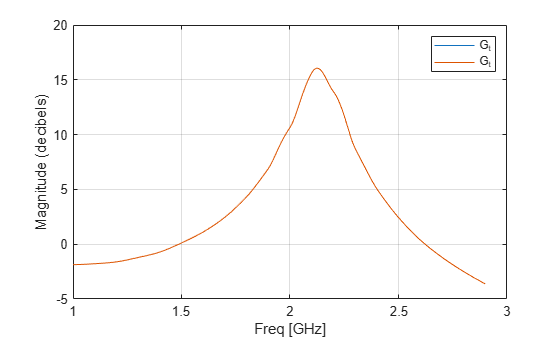# analyze

Analyze RFCKT object in frequency domain

## Syntax

``analyze(rfcktobject,frequency)``
``analyze(rfcktobject,frequency,zl,zs,zo,aperture)``
``analyze(rfcktobject,frequency,condition,value)``

## Description

example

````analyze(rfcktobject,frequency)` calculates the following rfckt data at the specified frequency values: Circuit network parametersNoise figureOutput third-order intercept pointPower dataPhase noiseVoltage standing-wave ratioPower gainGroup delayReflection coefficientsStability dataTransfer function ```
````analyze(rfcktobject,frequency,zl,zs,zo,aperture)` calculates the circuit data specified frequency values with optional arguments such as load impedance, source impedance, reference impedance and aperture.```
````analyze(rfcktobject,frequency,condition,value)` calculates the circuit data at the specified frequency values and operating conditions for the `circuitdata` object. For more information to set conditions and values, see `setop` function. NoteWhen you specify condition/value pairs, the `analyze` method changes the object's values to match your specification. ```

## Examples

collapse all

Create and analyze a two-wire network object.

``` tx1=rfckt.twowire('Radius',7.5e-4); analyze(tx1,1.9e9)```
```ans = rfckt.twowire with properties: Radius: 7.5000e-04 Separation: 0.0016 MuR: 1 EpsilonR: 2.3000 LossTangent: 0 SigmaCond: Inf LineLength: 0.0100 StubMode: 'NotAStub' Termination: 'NotApplicable' nPort: 2 AnalyzedResult: [1x1 rfdata.data] Name: 'Two-Wire Transmission Line' ```

This example shows how to analyze an RF amplifier at different reference impedances.

Assign the load and the source impedances.

```zl = 50 - 50*1i; zs = 200 + 50*1i;```

Create two amplifier circuits with the same Touchstone® file.

```circuit50 = read(rfckt.amplifier,'default.s2p'); circuit75 = read(rfckt.amplifier,'default.s2p');```

Analyze the amplifier circuits at two different reference impedance, `50` and `75` ohms.

```analyzed_circuit50 = analyze(circuit50, circuit50.NetworkData.Freq, zl, zs,50); analyzed_circuit75 = analyze(circuit75, circuit50.NetworkData.Freq, zl, zs,75);```

Plot S21 for the two amplifier circuits.

```figure(30); plot(analyzed_circuit50,'S21') hold on; plot(analyzed_circuit75,'S21')```Note that in this example two amplifier circuits derived from a same touchstone file at two different reference impedances produce two different S21 plot. This is because, the S-parameters are only dependent on the reference impedance, `z0`, and not on the source impedance, `zs` or the load impedance, `zl`.` `

The analyze function stores `zs` and `zl` in the amplifier and these impedances are used when a `zs` and `zl` dependent parameter is calculated. For example, plot transducer gain, `Gt` of the two amplifier circuits.

```figure(20); plot(analyzed_circuit50,'Gt') hold on; plot(analyzed_circuit75,'Gt')```Note that `Gt` is dependent on `zs`, `zl`, and `z0`. Hence for the two amplifier circuits derived from a same touchstone file with same `zs` and `zl` at `z0` of `50` and `75` ohms yields the same `Gt`.

## Input Arguments

collapse all

RFCKT object to analyze, specified as a object handle.

Example: ```amp = rfckt.amplifier```;`analyze(amp,frequency)`Analyzes the `rfckt.amplifier` object with handle `amp` at the specified frequency.

Data Types: `char` | `string`

Simulation frequencies, specified as a vector in hertz.

Example: `1.9e9`

Data Types: `double`

Load impedance, specified as a scalar in ohms.

Example: `40`

Data Types: `double`

Source impedance, specified as a scalar in ohms.

Example: `40`

Data Types: `double`

Reference impedance of S-parameters, specified as a real positive scalar or real positive vector in ohms. The length of this vector must be same as of frequency argument.

Example: `40`

Data Types: `double`

Value to determine two closely spaced frequencies at each simulation frequency for the calculation of group delay, specified as a positive scalar or a vector of same length as simulation frequencies. If the `aperture` is not specified, it will be determines based on the simulation frequencies.

Example: `40`

Data Types: `double`

## Version History

Introduced before R2006a